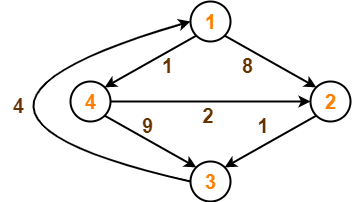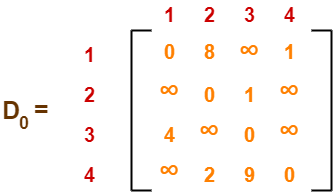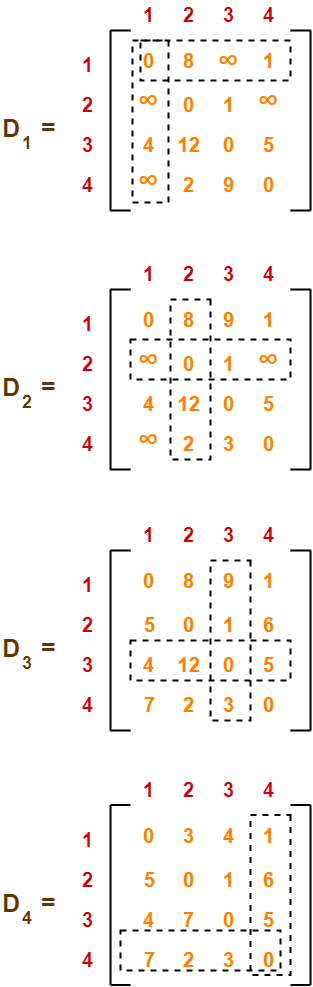Floyd Warshall Algorithm-

• Floyd Warshall Algorithm is a famous algorithm.
• It is used to solve All Pairs Shortest Path Problem.
• It computes the shortest path between every pair of vertices of the given graph.
• Floyd Warshall Algorithm is an example of dynamic programming approach.

Floyd Warshall Algorithm has the following main advantages-

• It is extremely simple.
• It is easy to implement.

Algorithm-

Floyd Warshall Algorithm is as shown below-

Create a |V| x |V| matrix               // It represents the distance between every pair of vertices as given
For each cell (i,j) in M do-
if i = = j
M[ i ][ j ] = 0                 // For all diagonal elements, value = 0
if (i , j) is an edge in E
M[ i ][ j ] = weight(i,j)       // If there exists a direct edge between the vertices, value = weight of edge
else
M[ i ][ j ] = infinity          // If there is no direct edge between the vertices, value = ∞
for k from 1 to |V|
for i from 1 to |V|
for j from 1 to |V|
if M[ i ][ j ] > M[ i ][ k ] + M[ k ][ j ]
M[ i ][ j ] = M[ i ][ k ] + M[ k ][ j ]

Time Complexity-

• Floyd Warshall Algorithm consists of three loops over all the nodes.
• The inner most loop consists of only constant complexity operations.
• Hence, the asymptotic complexity of Floyd Warshall algorithm is O(n3).
• Here, n is the number of nodes in the given graph.

When Floyd Warshall Algorithm Is Used?

• Floyd Warshall Algorithm is best suited for dense graphs.
• This is because its complexity depends only on the number of vertices in the given graph.
• For sparse graphs, Johnson’s Algorithm is more suitable.

Problem-

Consider the following directed weighted graph-Using Floyd Warshall Algorithm, find the shortest path distance between every pair of vertices.

Solution-

Step-01:

• Remove all the self loops and parallel edges (keeping the lowest weight edge) from the graph.
• In the given graph, there are neither self edges nor parallel edges.

Step-02:

• Write the initial distance matrix.
• It represents the distance between every pair of vertices in the form of given weights.
• For diagonal elements (representing self-loops), distance value = 0.
• For vertices having a direct edge between them, distance value = weight of that edge.
• For vertices having no direct edge between them, distance value = ∞.

Initial distance matrix for the given graph is-Step-03:

Using Floyd Warshall Algorithm, write the following 4 matrices-To learn how to write these matrices, watch this video here.

The last matrix D4 represents the shortest path distance between every pair of vertices.

Remember-

• In the above problem, there are 4 vertices in the given graph.
• So, there will be total 4 matrices of order 4 x 4 in the solution excluding the initial distance matrix.
• Diagonal elements of each matrix will always be 0.

To gain better understanding about Floyd Warshall Algorithm,

Watch this Video Lecture

Next Article- Knapsack Problem

Get more notes and other study material of Design and Analysis of Algorithms.

Watch video lectures by visiting our YouTube channel LearnVidFun.